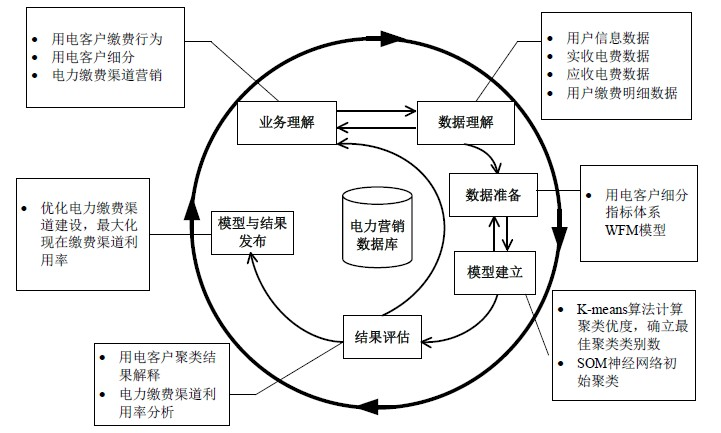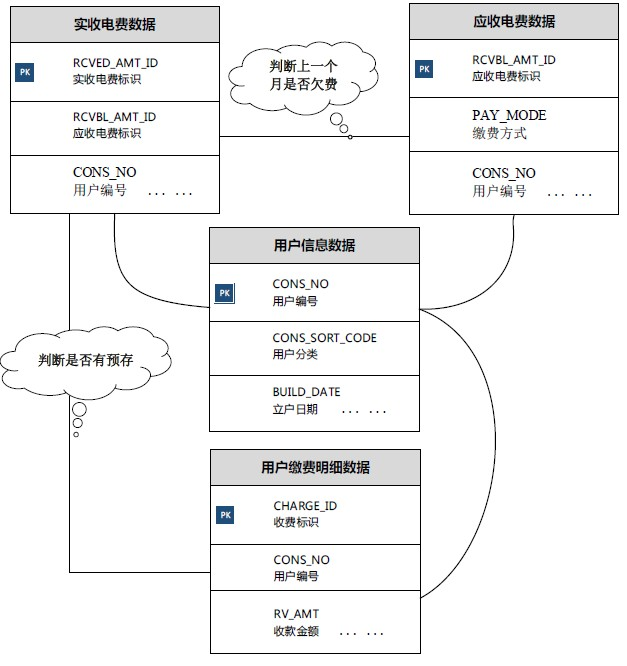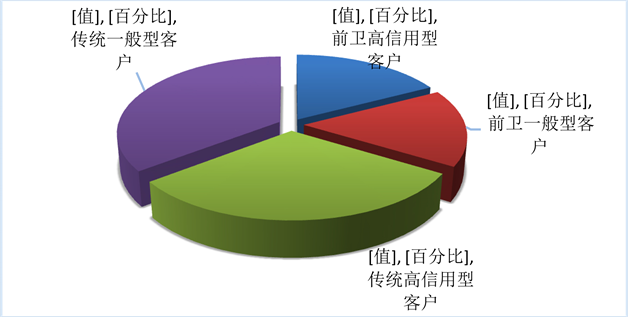﻿ 基于缴费行为的用电客户细分研究

# 基于缴费行为的用电客户细分研究Electricity Customer Segmentation Study Based on Payment Behavior

Abstract: It is effective to improve electricity companies’ efficiency of collecting electricity charge by subdividing the payment behavior of electricity customers and adopting the improvement measures targeted. Based on the traditional RFM model, this paper proposes an index system and customer segmentation method which is suitable for the power customer system. Firstly, the WFM index system based on the electricity customer’s payment behavior is established. Then, K-means clustering method is used to obtain the optimal number of cluster with the best clustering goodness. The SOM neural network is used to cluster the customers, and the attributes of each group are compared with the overall attributes. So, the customer segmentation results are obtained. Finally, this method is applied to the detail data of electricity customer in Ningcheng County, Chifeng City, Inner Mongolia. Four different types of customer groups are extracted, and the corresponding marketing strategies of payment channels are put forward respectively. So, the feasibility and validity of this method are verified.

1. 引言

2. 用电客户缴费行为细分指标体系

1) W指标为用电客户不同的缴费方式，具体有W1、W2、W3和W4三种方式。W1指标为电力机构坐收使用频次，该指标在一定程度上反映了客户的缴费习惯，该习惯较为传统且使用频次高。W2指标为电Figure 1. The process of subdivision and mining of electricity customers’ payment behaviorFigure 2. Relationship between data tablesTable 1. Comparison between RFM model and WFM model

2) F指标分为实际缴费次数和预存缴费次数。涂莹等将用电客户缴费时间纳入电费回收风险模型中进行研究，并证明了其相关关系  。本研究中AF指标为实际缴费次数，虽然电费每月都会有收缴，但是客户的缴费频率却不是每月一次，该指标在一定程度上能够反映客户的缴费行为和缴费积极性。PF指标为预存缴费次数，具体指客户实际缴纳金额大于当月应收金额，该指标在一定程度上能够反映客户的缴费行为和缴费信用度。

3) M指标为缴费金额，本文所选客户均为低压居民，电价基本趋于稳定，所以电费的高低可以在一定程度上反映客户的用电稳定性。

3. 基于SOM神经网络的用电客户聚类

3.1. 聚类优度分析

${X}^{*}=\frac{x-\mathrm{min}}{\mathrm{max}-\mathrm{min}}$ (1)

$p=\frac{\text{between_ss}}{\text{within_ss}}$ (2)

P越大代表聚类优度越高，即聚类结果越好。根据实际情况，选取相对较高的聚类优度P*所对应的的聚类类别数K*，对实际数据进行分析。

3.2. SOM神经网络聚类算法

SOM神经网络的网络结构比较简单，由输入层和竞争层构成，输入层有N个神经元；竞争层有M个神经元。其中N为指标体系中所选指标数，M为聚类类别数，即M = K*。网络的连接权重为

$\left\{{w}_{ij}\right\},i=1,2,\cdots ,N;j=1,2,\cdots ,M$ ；约束条件为： $\underset{i=1}{\overset{N}{\sum }}{w}_{ij}=1$ 。网络的P个二值(0、1)输入学习模式为： ${A}_{k}=\left({a}_{1}^{k},{a}_{2}^{k},{a}_{3}^{k},\cdots ,{a}_{N}^{k}\right)$ ；与其对应的竞争层输出模式为： ${B}_{k}=\left({b}_{1}^{k},{b}_{2}^{k},{b}_{3}^{k},\cdots ,{b}_{N}^{k}\right)$ ，其中 $k=1,2,\cdots ,P$

Step 1：初始化，按约束条件 $\underset{i=1}{\overset{N}{\sum }}{w}_{ij}=1$ ，赋予 ${w}_{ij}$ [0, 1]内的随机值；

Step 2：任选P个模式中的一个模式 ${A}_{k}$ 提供给网络的输入层；

Step 3：计算竞争层各神经元的输入值 ${S}_{j}$${S}_{j}=\underset{i=1}{\overset{N}{\sum }}{w}_{ij}{a}_{ij},i=1,2,\cdots ,M$

Step 4：按“优胜劣汰”的原则以 ${S}_{j}\left(j=1,2,\cdots ,M\right)$ 中最大值所对应的神经元作为胜者，将其输出状态为1，而其它所有神经元的输出状态置为0，即： ${b}_{j}=1,{S}_{j}>{S}_{i},\left(i=1,2,\cdots ,M,i\ne j\right)$${b}_{i}=0,\left(i\ne j\right)$ ；若出现 ${S}_{j}={S}_{i}$ 的现象，则按统一约定取左边的神经元为获胜神经元；

Step 5：修正与获胜神经元相连的各连接权重，而其它所有连接权重保持不变 ${w}_{ij}={w}_{ij}+\Delta {w}_{ij}$ $\Delta {w}_{ij}=\eta \left(\frac{{a}_{i}^{k}}{m}-{w}_{ij}\right)$ 其中， $i=1,2,\cdots ,N,0<\eta <1$$\eta$ 为学习系数，一般为0.01~0.03，m为第k个学习模式向量 ${A}_{k}=\left({a}_{1}^{k},{a}_{2}^{k},{a}_{3}^{k},\cdots ,{a}_{N}^{k}\right)$ 中元素为1的个数；

Step 6：选取另一个学习模式，回到Step 3，直到P个学习模式全部提供给网络；

Step 7：返回Step 2，直到各连接权的调整量变得很小为止。

4. 应用实例

4.1. 数据预处理与模型参数设定

SOM神经网络的输入层有N个神经元；竞争层有M个神经元。本文中N = 7，分别为WFM模型中的七个细分指标，实验数据聚类类别数M = K* = 10，即将用电客户聚为10类。

4.2. 计算结果分析

SOM神经网络聚类分析共得到10类不同属性的客户群，每类客户群具体的用电客户数量如图4所示。其中，第三类(cluster 3)和第五类(cluster 5)的数量较多，均达到11,000个用户以上；第九类相对较少，只有3268个用户。可以直观看出，SOM神经网络聚类的十个客户群，在数量上存在一定的差异。为分析每个客户群具体指标的差异，采用比较每一个聚类群体细分指标均值和总体细分指标均值的变动情况来分析，具体数据分析如表3所示。Table 2. The distribution of WFM model indicators in application dataFigure 3. Selection of optimal number of clusteringFigure 4. Clustering results of SOM neural networkTable 3. Index analysis of SOM neural network clustering results

4.3. 营销策略建议

1) 对于传统一般型客户，电力公司应该采取积极主动的方式尽力争取，让这类群体意识到各类互联网缴费方式的便利性，同时，可以推出预存电费奖励机制，鼓励客户及时缴纳电费和预存电费，提高客Figure 5. Final classification results

2) 对于传统高信用型客户，电力公司应该抓住机会积极推广自助缴费终端、手机客户端缴费包括支付宝、电力E行、移动“和生活”、电信“翼支付”付等方式，让这部分客户多接触全新的缴费模式。同时，基于此类客户的历史缴费信用较好，可以给这类客户一定的欠费额度。

3) 对于前卫一般型客户，他们对于新兴事物的接受能力较强，电力公司在推出新的缴费方式时可以优先通过各种宣传方式通知他们试用。同时，也要告诉给他们一定的缴费和预存缴费的激励措施，确保电费收缴的及时性。

4) 对于前卫高信用型客户，从分类结果来看，此类客户不仅能够快速接受新兴缴费方式而且信用度较高，预存电费的频率高，对传统电力机构坐收资源的占用量少。基于此，电力公司可以采取保持其积极性的措施，如新客户端试用免单、预存电费年终积分兑换等形式，维持该类客户的积极性和信用度。

5. 结束语

NOTES

*通讯作者。

 田鹤. 电力多渠道缴费理论及缴费模式优化策略分析[J]. 科技创新导报, 2012(35): 41-43.

 王珣. 电力营销组织管理创新方法探索[J]. 电力需求侧管理, 2016, 18(5): 38-40.

 Antreas, D. (2000) Athanassopoulos. Customer Satisfaction Cues to Sup-port Market Segmentation and Explain Switching Behavior. Journal of Business Research, 47, 191-207.
https://doi.org/10.1016/S0148-2963(98)00060-5

 赵铭, 李雪, 李秀婷, 等. 基于聚类分析的商业银行基金客户的分类研究[J]. 管理评论, 2013, 25(7): 38-44.

 褚格林. 基于聚类模型的电信客户细分研究[J]. 统计与决策, 2014(8): 176-179.

 郭崇慧, 赵作为. 基于客户行为的4S店客户细分及其变化挖掘[J]. 管理工程学报, 2015, 29(4): 18-26.

 蔡淑琴, 蒋士淼, Olle, G.D., 等. 基于在线客户评论的客户细分研究[J]. 管理学报, 2015, 12(7): 1059-1063.

 胡桂莉, 喻昕, 黄秋婵. 基于SOM神经网络的移动客户细分研究[J]. 微型机与应用, 2015, 34(23): 51-54.

 帅斌, 邓绍蔚, 黄丽霞. 基于改进RFM模型的铁路快捷货运客户市场细分方法[J]. 铁道科学与工程学报, 2014, 11(1): 112-117.

 黄聪, 王东. 基于RFM分析模式与马尔可夫链的客户行为预测模型研究[J]. 情报杂志, 2009, 28(S2): 143-146.

 宋才华, 蓝源娟, 范婷, 等. 电力企业客户细分模型研究[J]. 现代电子技术, 2014(2): 91-94.

 赵莉, 候兴哲, 胡君, 等. 基于改进K-均值算法的海量智能用电数据分析[J]. 电网技术, 2014, 38(10): 2715-2720.

 胡晓雪, 赵嵩正, 吴楠, 等. 基于SOM-DB-PAM混合聚类算法的电力客户细分[J]. 计算机工程, 2015, 41(10): 295-301.

 刘晓焜. 基于电力缴费行为细分的服务提升研究[J]. 中国科技信息, 2014(23): 185-186.

 Kohonen, T. (1990) The Self-Organizing Map. Proceedings of the IEEE, 78, 1464-1480.
https://doi.org/10.1109/5.58325

 Vesanto, J. and Alhoniemi, E. (2000) Clustering of the Self-Organizing Map. IEEE Trans-actions on Neural Networks, 11, 586-600.
https://doi.org/10.1109/72.846731

 George, C. (2013) Hadjinicola. Product Posi-tioning Using a Self-Organizing Map and the Rings of Influence. Decision Sciences, 44, 431-461.
https://doi.org/10.1111/deci.12020

 涂莹, 林士勇, 欧阳柳, 等. 基于市场细分的逻辑回归模型在电费回收风险预测中的应用研究[J]. 电力需求侧管理, 2016, 18(4): 46-49.

Top